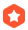# matlab符号运算函数wpf8898 2022-06-16 21:21:35加精

定义变量      syms

内联函数   f=inline('5*x+1','x')

f=@(x)5*x+3

M函数

solve

用符号变量替换变量  subs(f，[    ])

因式分解  factor函数，调用格式为：factor(s)，对符号表达式s进行因式分解

合并同类项。使用collect函数，调用格式为：collect(s)

符号表达式的展开。expand，调用格式为：expand(s)，对符号表达式s进行展开

求反函数      g=finverse(f,v)  求f的反函数，变量为v

compose(f,g)，计算复合函数f(g(x))

求极限          limit(f,x,0)

y=dsolve('equation','x')     %equation指符号微分方程，x为符号变量

求导 diff(S, ’x’, n)对表达式中指定符号x计算n阶导数。

Int(S, v, a, b) 这里a, b对积分区间进行了限定

格式美化。使用pretty函数，调用格式为：pretty(s)，使书写格式更加美观。

fplot()

ezplot()

...全文
39 2 打赏 收藏 举报

2 条回复matlab符号运算函数大全.pdf
matlab符号运算函数大全，讲述常用的matlab符号运算函数，包括solve等一系列运算函数matlab符号运算函数大全[定义].pdf
matlab符号运算函数大全[定义].pdfMATLAB符号运算.doc
MATLAB编程符号计算 行命令a=sym('pi','d')，则对于变量a的描述 分别使用 sym 和syms创建符号表达式“sin(x)+cos(y)”matlab数学符号运算函数列表
%s为符号表达是，n为分子，,d为分母 PS：无论s是什么，MATLAB会进行运算，使得s化为一个分式 3、符号表达式的因式分解与展开 factor(s); %对符号表示式分解分解因式 expands(s); %对s进行展开 collect(s); %对s...MATLAB符号运算小技巧

1.4w+

1. 智能科学是门槛很高的领域，您可以聊聊学习过程中的困惑或见解；
2. “万事配环境难”，您可以发布遇到的Bug或解决方案；
3. 谈谈职场面经，与同行信息共享、优势互补；
4. 分享自己或他人的优秀博文，为大家增长见识，不限领域；

• 恶意刷屏和广告；
• 措辞不文明甚至人身攻击；
• 其他违反国家法律和社会道德的行为